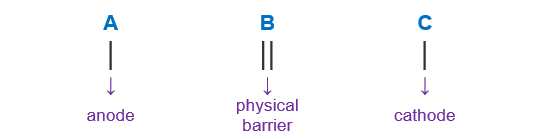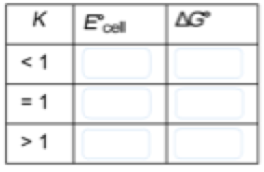# Problem: For the following battery Cd(s) | CdCl2(aq) || Cl- (aq) | Cl2(l)| C(s) (a) Write the reduction half-reaction occuring at the C(s) electrode (Include physical stales of reactants and products)C(s) electrode_________________   E degree =1.4 V(b) From which electrode will electrons flow from the battery into a circuit?a) Cd(s) electrode b) C(s) electrode (c) Calculate the mass of Cl2 consumed if the battery delivers a constant current of 617 A for 83.0 min.Complete this table relating the values of E°cell and  ΔG° to K.&gt;0 ,  =0, &lt;0

###### FREE Expert Solution

a.↓ E° → oxidation → anode

↑ E° → reduction → cathode

Oxidation:       Cd(s)  Cd2+ (aq) + 2 e-

Reduction:       Cl2 (l) + 2 e-⇌ 2 Cl-(aq)

b. Anode → electrons are generated via oxidation → electrons will flow from anode to cathode

Anode is Cd(s)

79% (110 ratings)###### Problem Details

For the following battery Cd(s) | CdCl2(aq) || Cl- (aq) | Cl2(l)| C(s)

(a) Write the reduction half-reaction occuring at the C(s) electrode (Include physical stales of reactants and products)

C(s) electrode_________________   E degree =1.4 V

(b) From which electrode will electrons flow from the battery into a circuit?

a) Cd(s) electrode

b) C(s) electrode

(c) Calculate the mass of Cl2 consumed if the battery delivers a constant current of 617 A for 83.0 min.

Complete this table relating the values of E°cell and  ΔG° to K.>0 ,  =0, <0